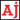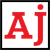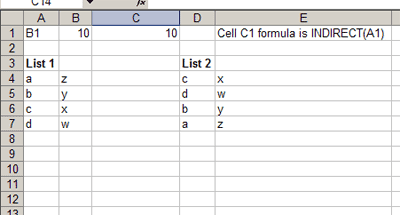# .css-t1tid{-webkit-text-decoration:none;text-decoration:none;background-image:none;text-shadow:none;}Reviews. Info. Tutorials.

# Using INDIRECT to dynamically reference a cell in Excel

Learn how to use the INDIRECT function to build cell references.
May 23, 2012

If you have ever wanted to use text to reference a cell then you will be interested to know how the `INDIRECT` function in Excel can help you out.

## INDIRECT function

The `INDIRECT` function accepts two parameters, but only one is required and truthfully, you'll never use the second parameter. The parameter you will be using is `ref_text`. It is the reference to a cell as a text string.

## Examples

To give you an idea of how it works, take the following example spreadsheet:Figure 1: Using the INDIRECT function

### Example 1

`=INDIRECT("B5")` will reference the cell at B5.

### Example 2

Say you type 'B1' in cell A1 and you enter the number 10 in cell B1. In cell C1 you enter the formula `=INDIRECT(A1)`, the result will be the number 10. It works that way because `INDIRECT` will take the text in cell A1 as the cell reference which in this case is 'B1' and the value in cell B1 is 10.

## Useful applications of INDIRECT

The most useful application for this function is when you want to build a cell reference from text and/or numbers. For example, if you want to match values in one list to another and then return the value in the cell adjacent to the search list. Let's see how this would play out in Figure 1.

There is List 1 and List 2. The values in column A and B have been typed in. In column D the values are typed. The formula used in cell E4 is `=INDIRECT("B" & (MATCH(D4,A\$4:A\$7,0)+3))`.

The formula looks for a match of the letter c (cell D4) in the list in column A and returns it's relative location if it finds a match (I added 3 because the list starts at row 4). So the match function will return the relative location and we want to know the value adjacent to that cell which is in column B. Using `INDIRECT` we can construct the cell reference by adding the text `"B"` and the result from the `MATCH` function. It's that simple, just use & to piece the parts together.